How to Calculate and Solve for Bulk Modulus of Elasticity (E) | Rock Mechanics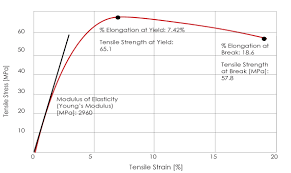The image above represents bulk modulus of elasticity (E).

To compute for bulk modulus of elasticity (E), two essential parameters are needed and these parameters are young’s modulus (E) and Poisson’s ratio (v).

The formula for calculating the bulk modulus of elasticity (E):

k = E / 3(1 – 2v)

Where:

k = Bulk Modulus of Elasticity (E)
E = Young’s Modulus
v = Poisson’s Ratio

Let’s solve an example;
Find the bulk modulus of elasticity (E) when the young’s modulus is 66 and the Poisson’s ratio is 58.

This implies that;

E = Young’s Modulus = 66
v = Poisson’s Ratio = 58

k = E / 3(1 – 2v)
k = 66 / 3(1 – 2(58))
k = 66 / 3(1 – 116)
k = 66 / 3(-115)
k = 66 / -345
k = -0.19

Therefore, the bulk modulus of elasticity (E) is -0.19.

Calculating the Young’s Modulus when the Bulk Modulus of Elasticity (E) and the Poisson’s Ratio is Given.

E = k (3 – 6v)

Where:

E = Young’s Modulus
k = Bulk Modulus of Elasticity (E)
v = Poisson’s Ratio

Let’s solve an example;
Find the young’s modulus when the bulk modulus of elasticity (E) is 22 and the Poisson’s ratio is 18.

This implies that;

k = Bulk Modulus of Elasticity (E) = 22
v = Poisson’s Ratio = 18

E = k (3 – 6v)
E = 22 (3 – 6(18))
E = 22 (3 – 108)
E = 22 (-105)
E = -2376

Therefore, the young’s modulus is -2376.

Calculating the Poisson’s Ratio when the Bulk Modulus of Elasticity (E) and the Young’s Modulus is Given.

v = -(E – 3k / 6k)

Where:

v = Poisson’s Ratio
k = Bulk Modulus of Elasticity (E)
E = Young’s Modulus

Let’s solve an example;
Find the Poisson’s ratio when the bulk modulus of elasticity (E) is 12 and the young’s modulus is 40.

This implies that;

k = Bulk Modulus of Elasticity (E) = 12
E = Young’s Modulus = 40

v = -(E – 3k / 6k)
v = -(40 – 3(12) / 6(12))
v = -(40 – 36 / 72)
v = -(4 / 72)
v = -(0.055)
v  = -0.055

Therefore, the Poisson’s ratio is -0.055.

Nickzom Calculator – The Calculator Encyclopedia is capable of calculating the bulk modulus of elasticity (E).

To get the answer and workings of the bulk modulus of elasticity (E) using the Nickzom Calculator – The Calculator Encyclopedia. First, you need to obtain the app.

You can get this app via any of these means:

To get access to the professional version via web, you need to register and subscribe for NGN 1,500 per annum to have utter access to all functionalities.
You can also try the demo version via https://www.nickzom.org/calculator

Android (Paid) – https://play.google.com/store/apps/details?id=org.nickzom.nickzomcalculator
Android (Free) – https://play.google.com/store/apps/details?id=com.nickzom.nickzomcalculator
Apple (Paid) – https://itunes.apple.com/us/app/nickzom-calculator/id1331162702?mt=8
Once, you have obtained the calculator encyclopedia app, proceed to the Calculator Map, then click on Geology under Add-on.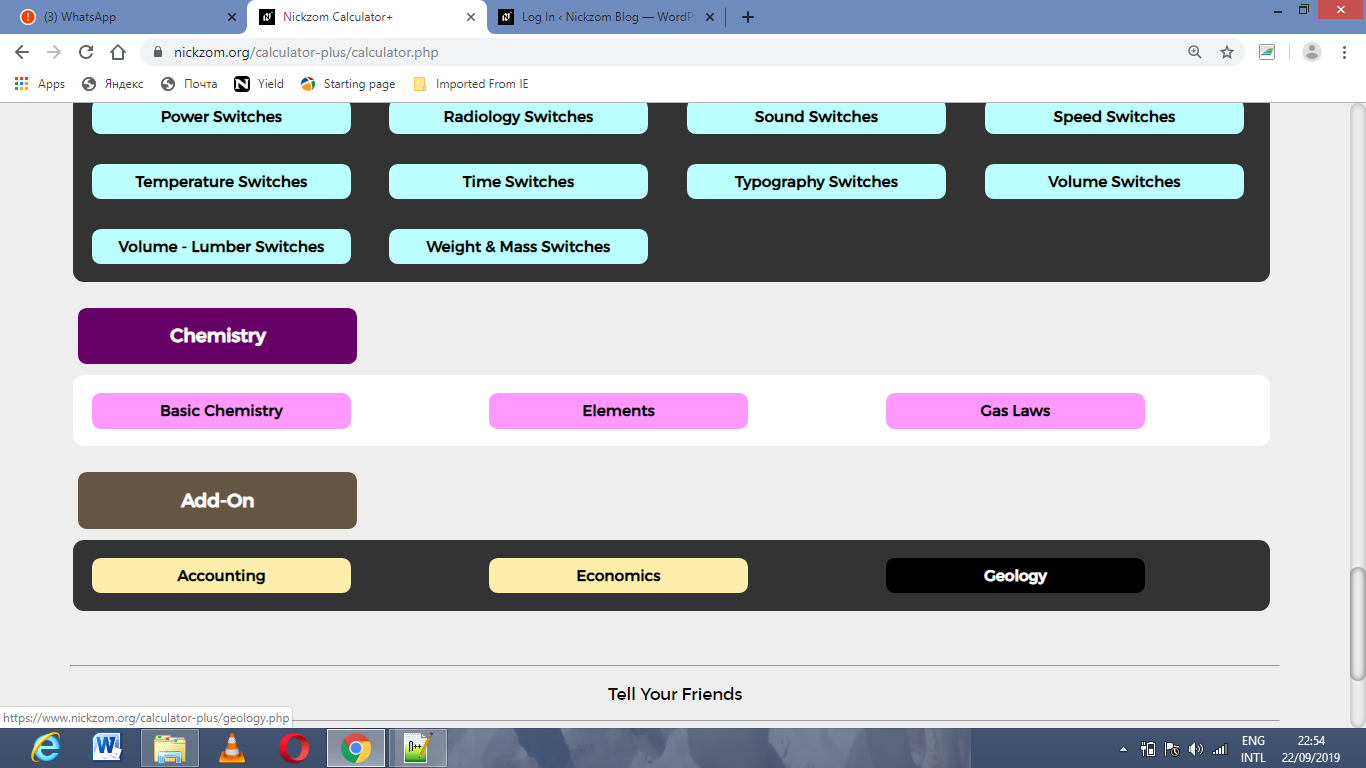Now, Click on Rock Mechanics under GeologyNow, Click on Bulk Modulus of Elasticity (E) under Rock Mechanics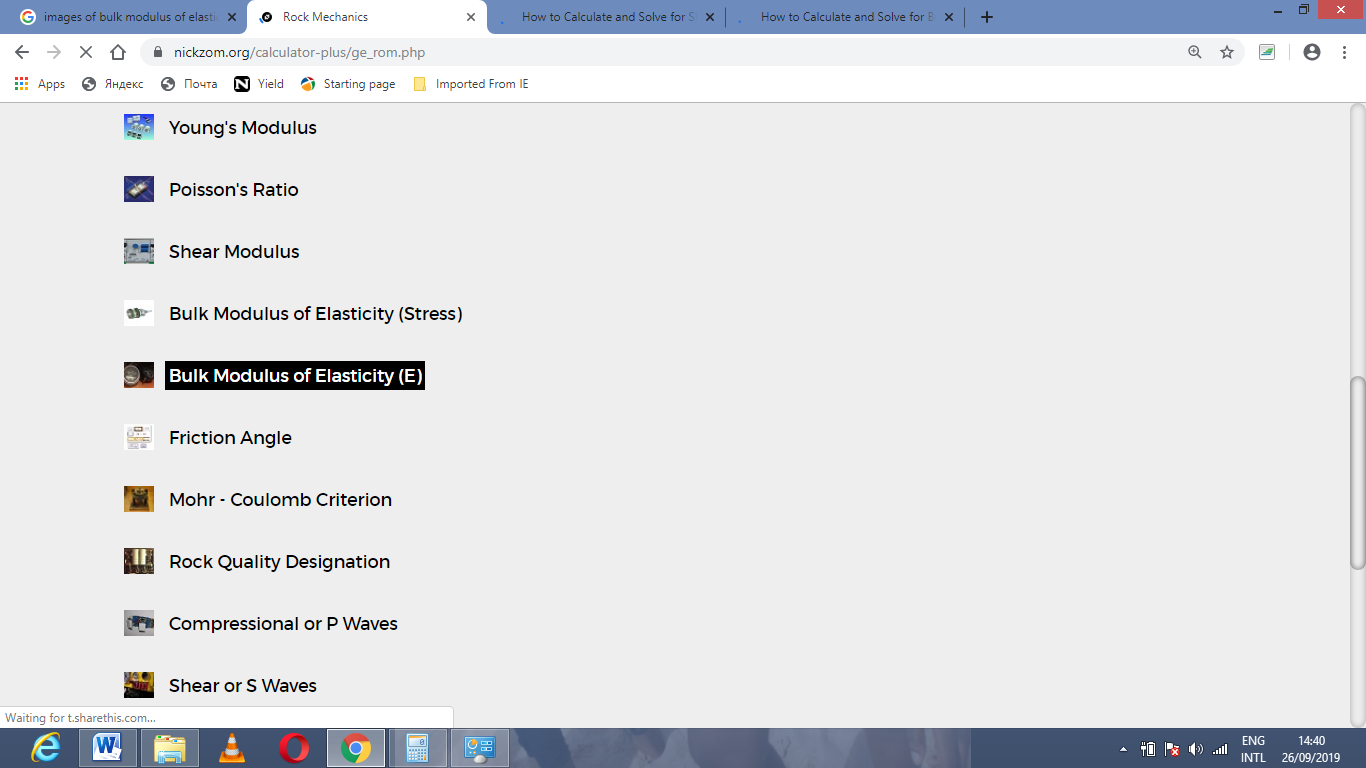The screenshot below displays the page or activity to enter your values, to get the answer for the bulk modulus of elasticity (E) according to the respective parameters which are the young’s modulus (E) and Poisson’s ratio (v).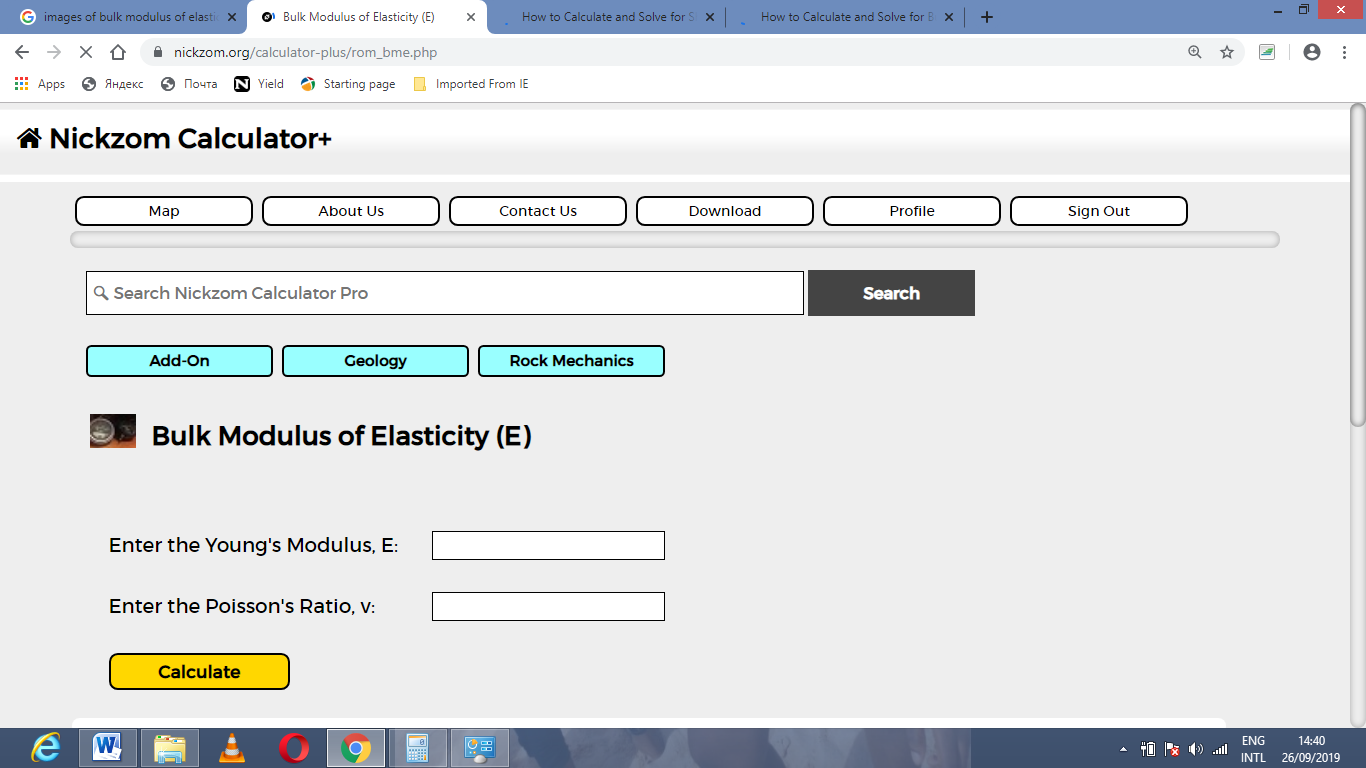Now, enter the values appropriately and accordingly for the parameters as required by the young’s modulus (E) is 66 and Poisson’s ratio (v) is 58.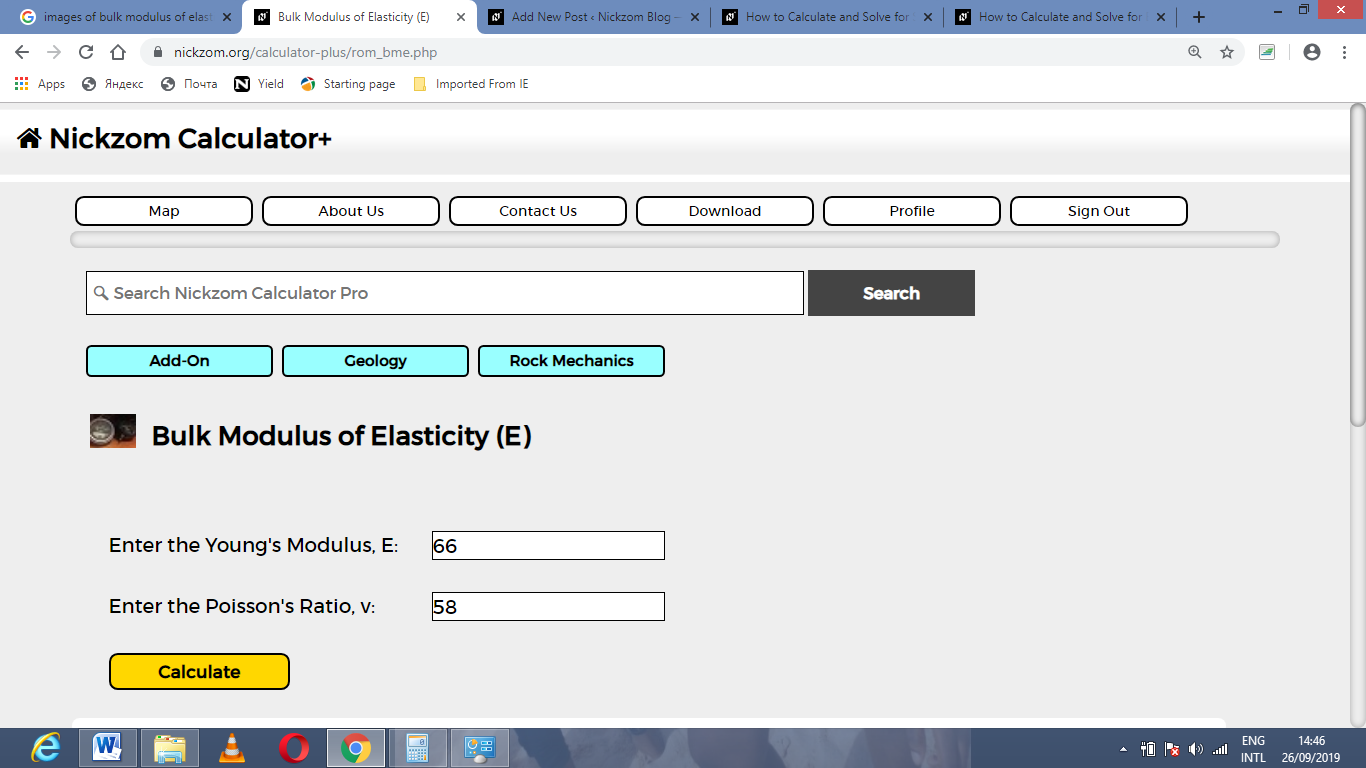Finally, Click on Calculate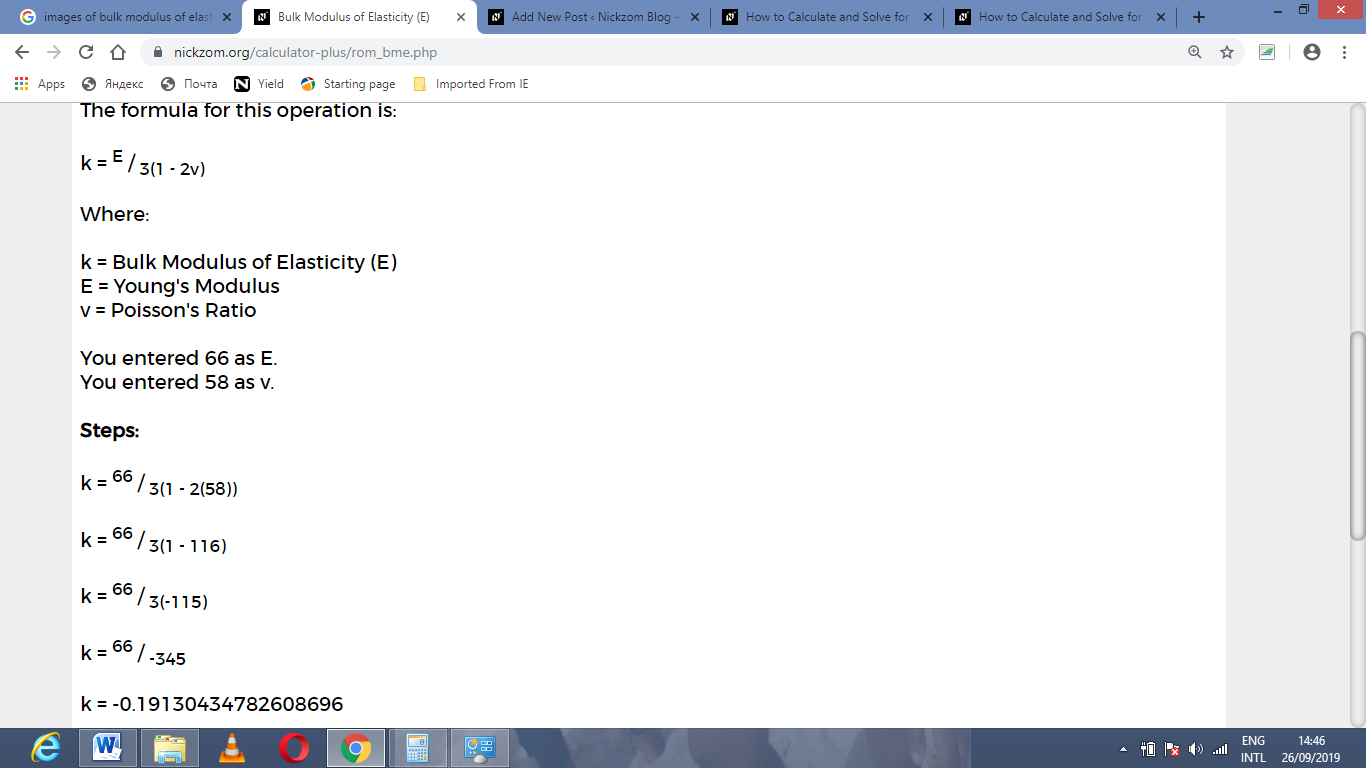As you can see from the screenshot above, Nickzom Calculator– The Calculator Encyclopedia solves for the bulk modulus of elasticity (E) and presents the formula, workings and steps too.